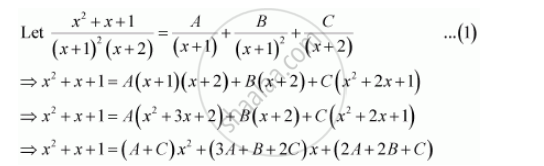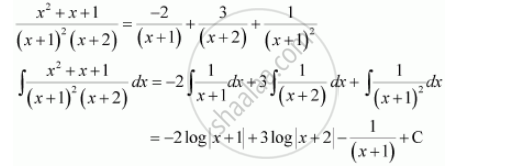Share

# Integrate the Functions (X^2 + X + 1)/((X + 1)^2 (X + 2)) - CBSE (Commerce) Class 12 - Mathematics

ConceptIntegration as an Inverse Process of Differentiation

#### Question

Integrate the functions (x^2 + x + 1)/((x + 1)^2 (x + 2))

#### SolutionEquating the coefficients of x2x,and constant term, we obtain

A + C = 1

3A + B + 2= 1

2A + 2B + C = 1

On solving these equations, we obtain

A = −2, B = 1, and C = 3

From equation (1), we obtainIs there an error in this question or solution?

#### Video TutorialsVIEW ALL 

Solution Integrate the Functions (X^2 + X + 1)/((X + 1)^2 (X + 2)) Concept: Integration as an Inverse Process of Differentiation.
S